## Nail tips exert tremendous pressures when they are hit by hammers because they exert a large force over a small area. What force (in N) must

Question

Nail tips exert tremendous pressures when they are hit by hammers because they exert a large force over a small area. What force (in N) must be exerted on a nail with a circular tip of 1.45 mm diameter to create a pressure of 2.33 ✕ 109 N/m2? (This high pressure is possible because the hammer striking the nail is brought to rest in such a short distance.)

in progress 0
6 months 2021-08-04T12:31:29+00:00 1 Answers 23 views 0

The  force is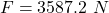Explanation:

From the question we are told that

The  diameter is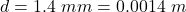The pressure is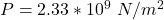Generally the radius is mathematically represented as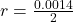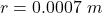The  cross-sectional area of the nail is mathematically represented as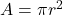=>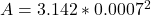=>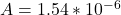Generally the pressure is mathematically represented as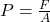=>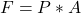=>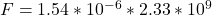=>